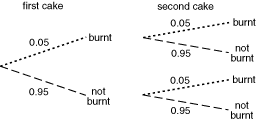Summary Article: tree diagram
From The Hutchinson Unabridged Encyclopedia with Atlas and Weather Guide

In probability theory, a branching diagram consisting only of arcs and nodes (but not loops curving back on themselves), which is used to establish probabilities. Each branch represents a possible outcome of one event. These possible outcomes are known as mutually exclusive events. The final probability depends on the path taken through the tree.

For example, when two cakes are baked the probability that one cake will not be burnt is 0.95. The tree diagram shows all the possible outcomes:The outcome of each cake can be described as an independent event as they have no effect on each other. Each path through the tree gives a different outcome. In this case there are four outcomes.

The dotted path gives the outcome of both cakes burning. The probabilities are multiplied to obtain this outcome:

Probability of both cakes burning = 0.05 × 0.05 = 0.0025

The dashed path gives the outcome of both cakes not burning:

Probability of both cakes not burning = 0.95 × 0.95 = 0.9025

The probability of one cake burning has two outcomes: (i) the first burnt and second not burnt, and (ii) the first not burnt and the second burnt. These probabilities are:

(i) 0.05 × 0.95 = 0.0475 and (ii) 0.95 × 0.05 = 0.0475

The two probabilities are added together to obtain the probability of one cake burning = 0.0475 + 0.0475 = 0.095.

The four probabilities should add up to 1.

Probabilities of independent events are always multiplied, characterized by and, for example, first cake burnt and second cake burnt. Probabilities of mutually exclusive events are always added, characterized by or, for example, first cake burnt or not burnt.

essays

Probability calculation using a tree diagram

Probability: tree diagrams© RM, 2018. All rights reserved.

### Related Articles

##### Full text Article TREE DIAGRAM
The SAGE Encyclopedia of Social Science Research Methods

In graph theory, a tree is defined as a completely connected graph without cycles. Like any other graph, a tree diagram consists of a set of...

##### Full text Article Probability
Encyclopedia of Survey Research Methods

In general, probability is a numerical representation of how likely is the occurrence of certain observations. Whenever an empirical...

##### Full text Article Laplace, Pierre Simon de (1749–1827),
Cambridge Dictionary of Philosophy

French mathematician and astronomer who produced the definitive formulation of the classical theory of probability. He taught at various schools in

See more from Credo